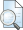# Jurnal Ilmiah PENGGUNAAN TEORI KEKONGRUENAN DALAM MEMPERKECIL RUANG PENCARIAN SOLUSI PERSAMAAN DIOPHANTINE x ^ 2 = y ^ 3 + 2185

No view

Jurnal Ilmiah PENGGUNAAN TEORI KEKONGRUENAN DALAM MEMPERKECIL RUANG PENCARIAN SOLUSI PERSAMAAN DIOPHANTINE x ^ 2 = y ^ 3 + 2185

USE OF CONTRUENTION THEORY IN SMALLING THE SEARCH FOR DIOPHANTINE EQUATION SOLUTIONS x 2 + 2185

from the Diophantine equation Ramanujan-Nagell x type 2 ] = y 3 + 2185. The purpose of this study was to find a search solution for the opportunities of diophantine Ramanujan-Nagell x 2  3 = y 3 + 2185 with x sub set of numbers odd numbers of members G 3 and G 4 while G 3 = {x∈ odd numbers | x≡1 mod 8} and G 4 = {x∈ odd numbers | x≡7 mod 8} with the method of calculating y into 4 cases, namely: FPB (y, 8) = 1, FPB (y, 8) = 2, FPB (y, 8) = 4, FPB (y, 8) = 8. The results of the study show that for x untukG 3 with FPB (y, 8) = 1, FPB (y, 8) = 4, FPB (y, 8) = 8, do not require integer solutions Meanwhile, for FPB (y, 8) = 2, although no conclusions have been obtained, the search space has been obtained for x and y by transforming x = 8b + 1, y = 4a – 2, moving a | b or b | a, then the equation Diophantine x 2  = y 3 + 2185 only has one pair of solutions, namely: (x, y) = (49.6), and for x∈G 4 with FPB (y, 8) = 1, FPB (y, 8) = 4, FPB (y, 8) = 8, FPB (y, 8) = 2 by transforming x = 8q + 7 , y = 4p – 2 for p | q or q | p does not have an integer solution. This study has succeeded in reducing the space for x and y.

Keywords [Era1945]: Euler's Theorem, Diophantine Equation, and Diophantine Ramanujan – Nagell